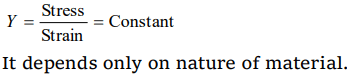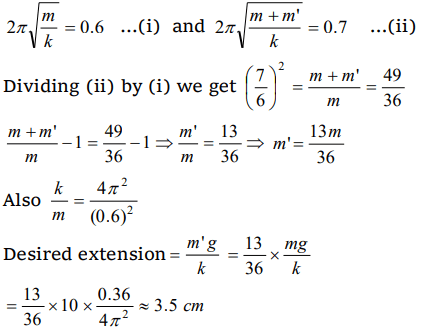## Mechanical Properties of Solids Questions and Answers Part-10

1. On applying a stress of $20 \times 10^{8}N\diagup m^{2}$   the length of a perfectly elastic wire is doubled. Its Young’s modulus will be
a) $40 \times 10^{8}N\diagup m^{2}$
b) $20 \times 10^{8}N\diagup m^{2}$
c) $10 \times 10^{8}N\diagup m^{2}$
d) $5 \times 10^{8}N\diagup m^{2}$

Explanation:2. When a uniform wire of radius r is stretched by a 2kg weight, the increase in its length is 2.00 mm. If the radius of the wire is r/2 and other conditions remain the same, the increase in its length is
a) 2.00 mm
b) 4.00 mm
c) 6.00mm
d) 8.00 mm

Explanation:3. The length of an elastic string is 'a' metre when the longitudinal tension is 4 N and 'b' metre when the longitudinal tension is 5 N. The length of the string in metre when the longitudinal tension is 9 N is
a) $a-b$
b) $5b-4a$
c) $2b-\frac{1}{4}a$
d) $4a-3b$

Explanation: Let L is the original length of the wire and K is force constant of wire.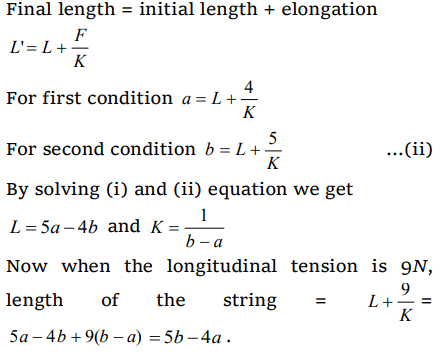4. Stress to strain ratio is equivalent to
a) Modulus of elasticity
b) Poission’s Ratio
c) Reyhold number
d) Fund number

Explanation: Modulus of elasticity

5. Which is correct relation
a) $Y<\sigma$
b) $Y>\sigma$
c) $Y=\sigma$
d) $\sigma=+1$

Explanation: $Y>\sigma$

6.If the interatomic spacing in a steel wire is 3.0 Å and $Y_{steel}=20 \times 10^{10}N\diagup m^{2}$     then force constant is
a) $6 \times 10^{-2}N\diagup \dot{A}$
b) $6 \times 10^{-9}N\diagup \dot{A}$
c) $4 \times 10^{-5}N\diagup \dot{A}$
d) $6 \times 10^{-5}N\diagup \dot{A}$

Explanation: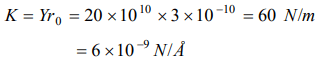7. A copper wire of length 4.0m and area of crosssection $1.2cm^{2}$ is stretched with a force of $4.8 \times 10^{3}N$   . If Young’s modulus for copper is $1.2 \times 10^{11}N\diagup m^{2}$    the increase in the length of the wire will be
a) 1.33 mm
b) 1.33 cm
c) 2.66 mm
d) 2.66 cm

Explanation: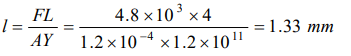8. A metal bar of length L and area of cross-section A is clamped between two rigid supports. For the material of the rod, its Young’s modulus is Y and coefficient of linear expansion is $\alpha$ . If the temperature of the rod is increased by $\triangle t^{0}C$   the force exerted by the rod on the supports is
a) $YAL\triangle t$
b) $YA\alpha\triangle t$
c) $\frac{YL\alpha\triangle t}{A}$
d) $Y\alpha AL\triangle t$

Explanation: $YA\alpha\triangle t$

9. According to Hook’s law of elasticity, if stress is increased, the ratio of stress to strain
a) Increases
b) Decreases
c) Becomes zero
d) Remains constant